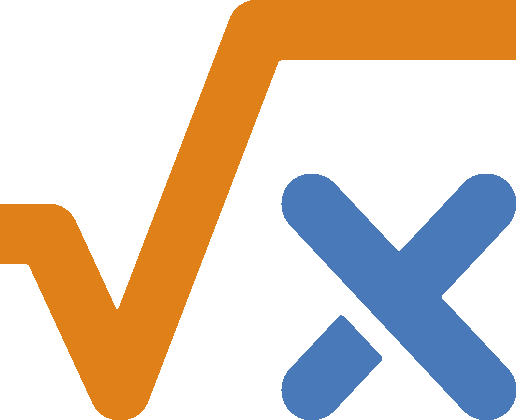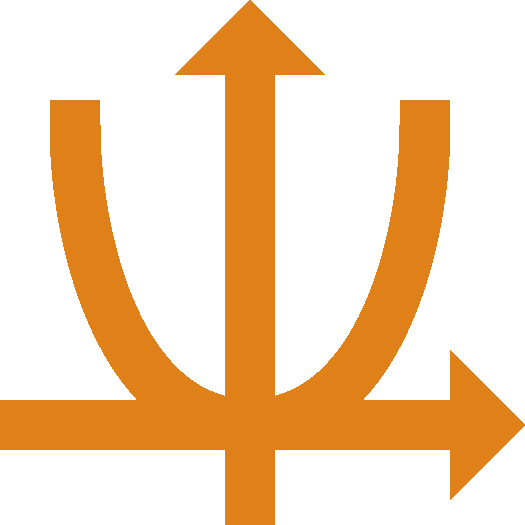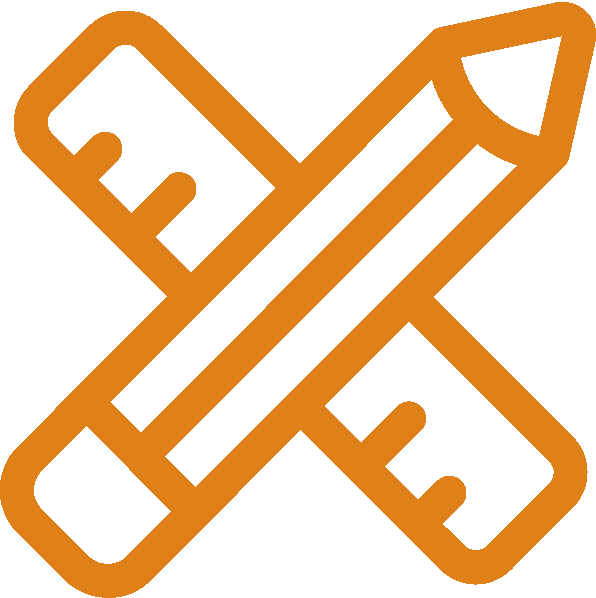Education programs | MIPT - Moscow Institute of Physics and Technology

## Research supervisor

•## Nikolay V. Bogachev

Candidate of Science,
PhD in Mathematics

Program description in Russian

## Supervisor’s research interests

I study geometric actions of groups on Riemannian manifolds and orbifolds. More precisely, let G be a discrete group of isometries of the hyperbolic Lobachevsky space H^n, then G is a hyperbolic lattice if the quotient hyperbolic orbifold H^n/G has finite volume. If G acts freely (ie is torsion free) then H^n/G is a hyperbolic manifold. If G is an arithmetic or quasi-arithmetic lattice then H^n/G is called so. Various examples of hyperbolic lattices are provided by the theory of hyperbolic reflection groups developed by Vinberg in 1967. A natural fundamental domain of a hyperbolic lattice generated by reflections in hyperplanes is a Coxeter polytope. In his fundamental paper, Vinberg described hyperbolic Coxeter polytopes in terms of its Coxeter diagrams (graphs) and proved a (quasi-)arithmeticity criterion for a hyperbolic reflection group.

## Supervisor’s specific requirementsAlgebra(group theory, rings and modules, number fields)Linear Algebra(vector spaces, linear maps, bilinear and quadratic forms)Topology(topology of R^n, topological spaces and manifolds)Geometry(convex polyhedra, smooth manifolds, Riemannian manifolds)

## Research highlights

The modern study of discrete groups combines:

• Algebraic approach
• Geometric approach
• Topological approach
• Combinatorial approach
• Number Theoretical approach

There are a lot of natural open questions in this theory.

## Main publications

• N. Bogachev, From geometry to arithmeticity of compact hyperbolic Coxeter polytopes, 2020, arXiv:2003.11944
• N. Bogachev, A. Kolpakov, On faces of quasi-arithmetic Coxeter polytopes, 2020, arXiv:2002.11445
• N. Bogachev, Classification of (1,2)-reflective anisotropic hyperbolic lattices of rank 4, Izvestiya Math, 2019, vol. 83:1, pp. 1—19.
• N. Bogachev, A. Perepechko, Vinberg’s algorithm for integral hyperbolic lattices, Math. Notes, 2019.